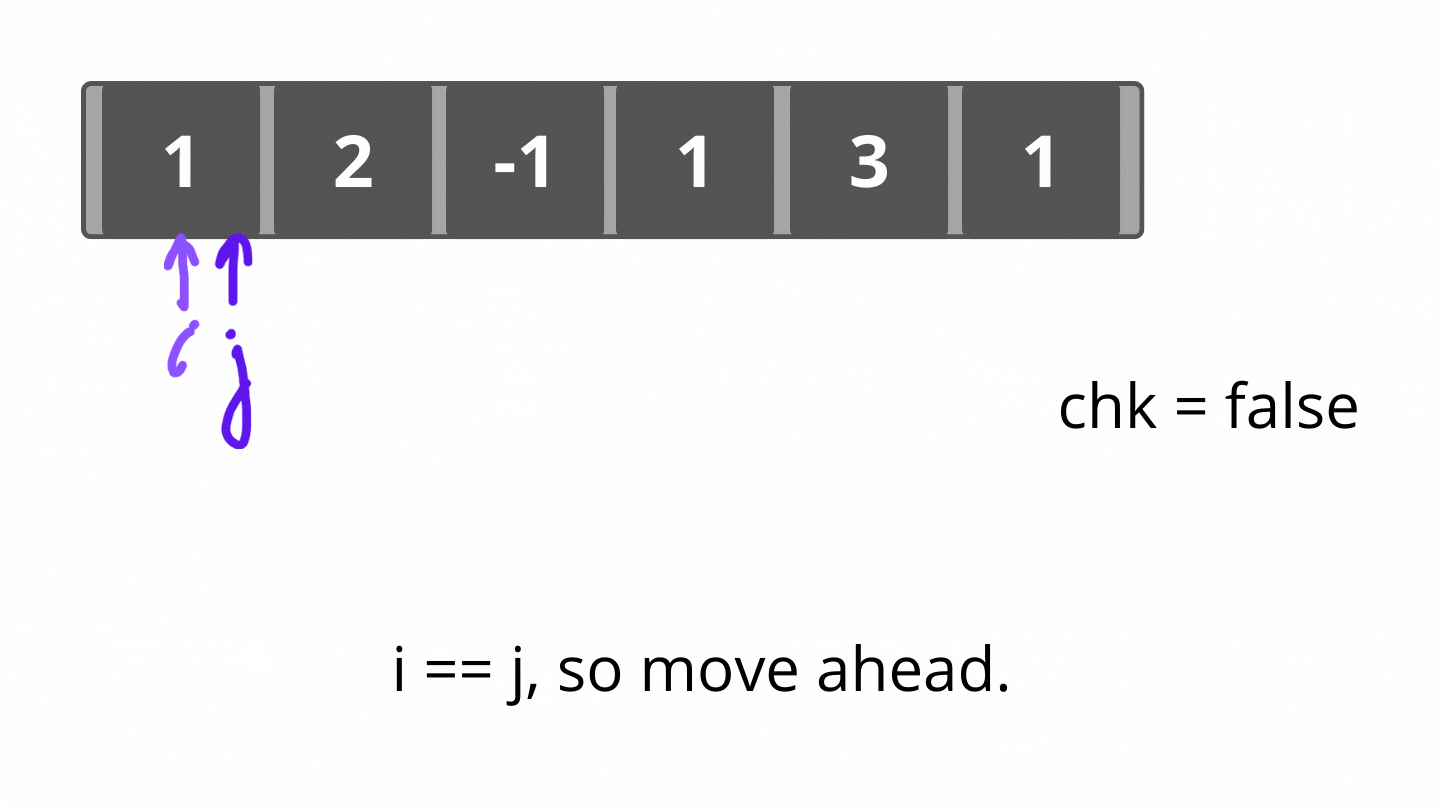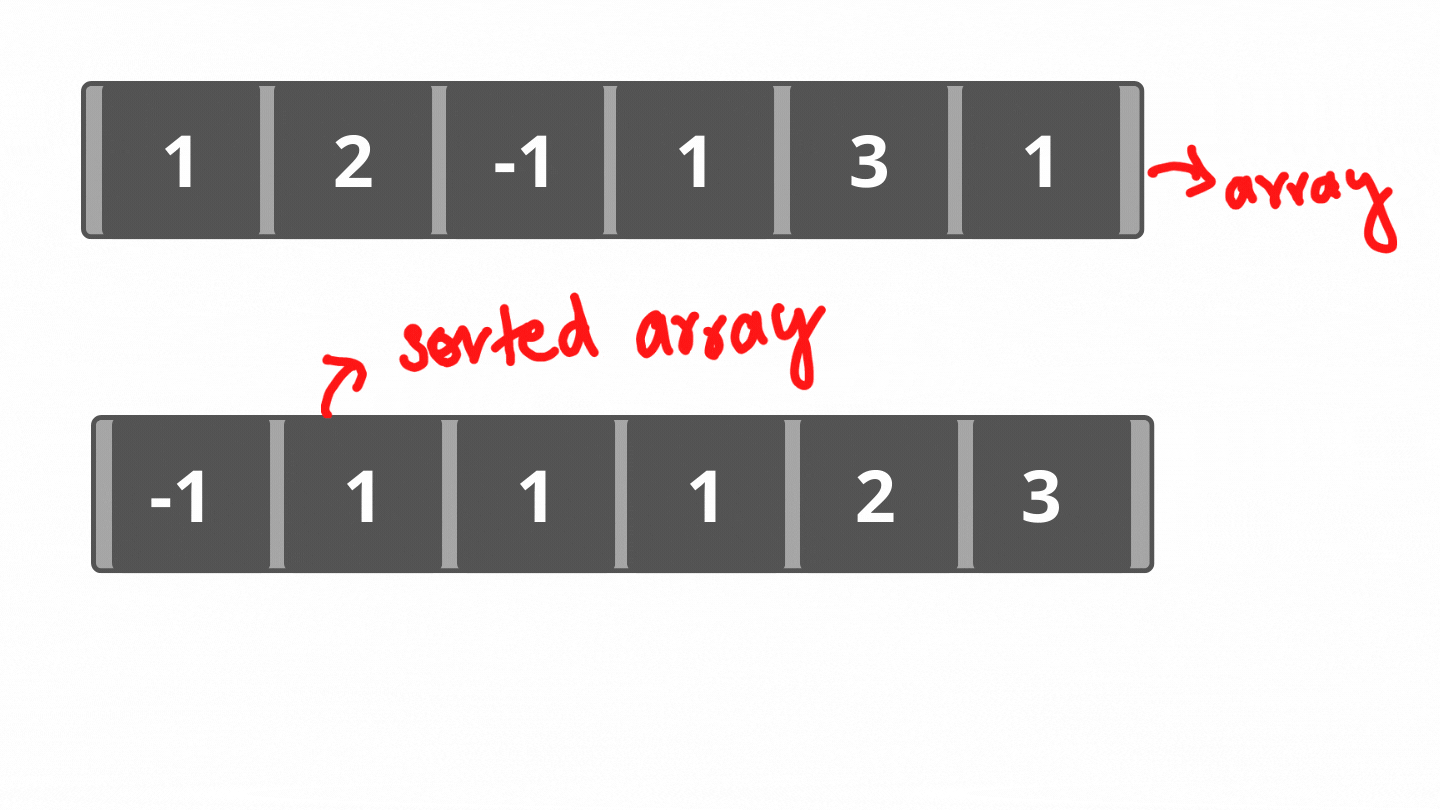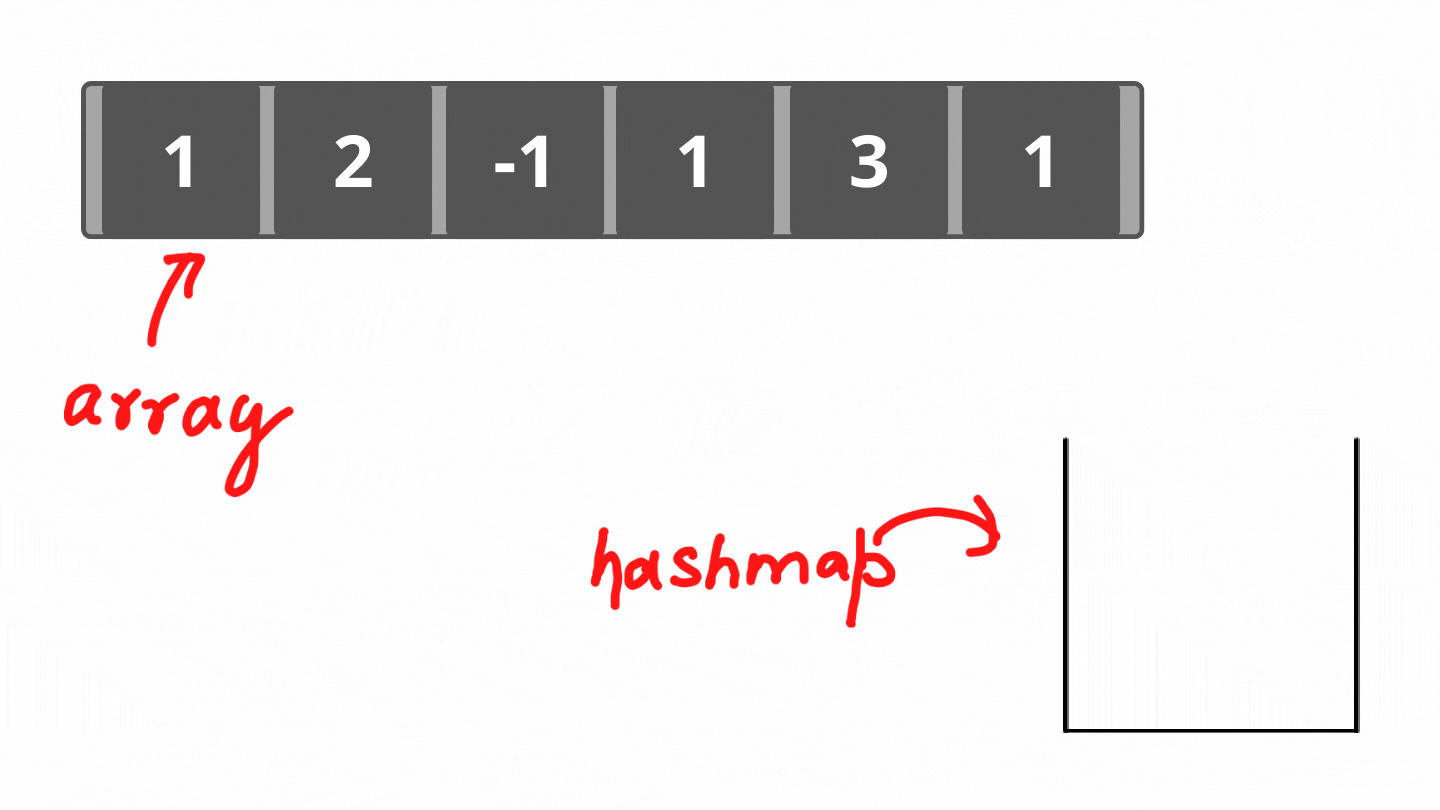# Find all the non-repeating elements in an array

Problem Statement: Find all the non-repeating elements for a given array. Outputs can be in any order.

Examples:

```Example 1:
Input:
Nums = [1,2,-1,1,3,1]
Output:
2,-1,3
Explanation:
1 is the only element in the given array which occurs thrice in the array. -1,2,3 occurs only once and hence, these are non-repeating elements of the given array.

Example 2:
Input:
Nums = [1,2,3]
Output:
1,2,3
Explanation:
All elements present in the array occur once. Hence, every element is non-repeating.```

Solution:

DisclaimerDon’t jump directly to the solution, try it out yourself first.

Solution 1: Brute-Force

Approach:

Following are the steps to the approach:

• Start iterating the array to pick an element.
• Use another nested loop to check if picked elements repeat in the array.
• If the inside nested loop reaches the end of the array for a picked element, it indicates it is a non-repeating element and we print it.
• If not, we move to pick another element.

Dry Run:Code:

## C++ Code

``````#include<bits/stdc++.h>
using namespace std;

void findNonRepeatingElement(vector<int>& nums) {
bool chk;
for(int i=0;i<nums.size();i++) {
chk = false;
for(int j=0;j<nums.size();j++) {
if(i!=j && nums[i] == nums[j]) {
chk = true;//to check if for current picked elements is repeating element
break;
}
}
//since chk is false only when no repeating element has occurred while traversal and reached the end of the array.
if(!chk) cout<<nums[i]<<" ";
}
}

int main() {
vector<int> nums = {1,2,-1,1,3,1};
cout<<"Non-repeating numbers are: "<<endl;
findNonRepeatingElement(nums);

return 0;
}
``````

Output:

Non-repeating numbers are:
2 -1 3

Time Complexity: O(N2)

Reason: We are using two loops nested.

Space Complexity: O(1)

Reason: No extra data structure is used for computation.

## Java Code

``````public class Main
{
static void findNonRepeatingElement(int nums[]) {
boolean chk;
for(int i=0;i<nums.length;i++) {
chk = false;
for(int j=0;j<nums.length;j++) {
if(i != j && nums[i] == nums[j]) {
chk = true;
break;
}
}
if(!chk) System.out.print(nums[i]+" ");
}
}
public static void main(String[] args) {
int nums[] = {1,2,-1,1,3,1};
System.out.println("Non-repeating numbers are: ");
findNonRepeatingElement(nums);
}
}
``````

Output:

Non-repeating numbers are:
2 -1 3

Time Complexity: O(N2)

Reason: We are using two loops nested.

Space Complexity: O(1)

Reason: No extra data structure is used for computation.

Solution 2: Using Sorting

Approach:

Following are the steps to the approach:

• Sort the given array.
• Iterate from 1st position to (n-2)th position(0-index).
• If the previous element, current element and next element are totally different. It is a non-repeating element.
• For edge cases, i.e, 0th and (n-1)th element. If the 0th element is not equal to the 1st element, then the 0th element is non-repeating. Similarly if (n-1)th element is not equal to (n-2)th element, (n-1)th element is non-repeating element.

Dry Run:Code:

## C++ Code

``````#include<bits/stdc++.h>
using namespace std;

void findNonRepeatingElement(vector<int>& nums) {
sort(nums.begin(),nums.end());//sorting array

//handling first element of the array.
if(nums != nums) cout<<nums<<" ";

for(int i=1;i<nums.size()-1;i++)
if(nums[i-1] != nums[i] && nums[i] != nums[i+1]) cout<<nums[i]<<" ";

//handling last element of the array
if(nums[nums.size()-2] != nums[nums.size()-1]) cout<<nums[nums.size()-1];
}

int main() {
vector<int> nums = {1,2,-1,1,3,1};
cout<<"Non-repeating numbers are: "<<endl;
findNonRepeatingElement(nums);

return 0;
}
``````

Output:

Non-repeating numbers are:
-1 2 3

Time Complexity: O(NlogN)+O(N)

Reason: O(NlogN) for sorting the array. O(N) for iteration.

Space Complexity: O(1)

Reason: No extra data structure was used.

## Java Code

``````import java.util.*;
public class Main
{
static void findNonRepeatingElement(int nums[]) {
Arrays.sort(nums);

if(nums != nums) System.out.print(nums+" ");

for(int i=1;i<nums.length-1;i++)
if(nums[i-1]!=nums[i] && nums[i]!=nums[i+1])
System.out.print(nums[i]+" ");

if(nums[nums.length-2] != nums[nums.length-1]) System.out.print(nums[nums.length-1]);
}
public static void main(String[] args) {
int nums[] = {1,2,-1,1,3,1};
System.out.println("Non-repeating numbers are: ");
findNonRepeatingElement(nums);

}
}
``````

Output:

Non-repeating numbers are:
-1 2 3

Time Complexity: O(NlogN)+O(N)

Reason: O(NlogN) for sorting the array. O(N) for iteration.

Space Complexity: O(1)

Reason: No extra data structure was used.

Solution 3: Using Maps

Approach:

Following are the steps to the approach:

• Declare a hashmap for storing elements as the key and their number of occurrences as value..
• Iterate through the array and store elements and their occurrences in the map.
• Print elements whose occurrences were equals to 1.

Dry Run:Code:

## C++ Code

``````#include<bits/stdc++.h>
using namespace std;

void findNonRepeatingElement(vector<int>& nums) {
// hashmap storing elements in the array as
// key and their occurrences as value.
unordered_map<int,int> hashMap;

for(auto i:nums) ++hashMap[i];
// if the count of elements equals to 1, it is a non-repeating element.
for(auto pairInMap:hashMap)
if(pairInMap.second == 1) cout<<pairInMap.first<<" ";
}

int main() {
vector<int> nums = {1,2,-1,1,3,1};
cout<<"Non-repeating numbers are: "<<endl;
findNonRepeatingElement(nums);

return 0;
}
``````

Output:

Non-repeating numbers are:
3 -1 2

Time Complexity: O(N)

Reason: Iterating once through the array once.

Space Complexity: O(N)

Reason: Map is used to insert elements.

## Java Code

``````import java.util.*;
import java.util.Map.Entry;
public class Main
{
static void findNonRepeatingElement(int nums[]) {
HashMap<Integer,Integer> hashMap = new HashMap<>();

for(int i:nums) {
if(hashMap.get(i) == null) hashMap.put(i,1);
else hashMap.put(i,hashMap.get(i)+1);
}

for(Entry<Integer,Integer> entry:hashMap.entrySet()) {
if(entry.getValue()==1)
System.out.print(entry.getKey()+" ");
}
}
public static void main(String[] args) {
int nums[] = {1,2,-1,1,3,1};
System.out.println("Non-repeating numbers are: ");
findNonRepeatingElement(nums);
}
}
``````

Output:

Non-repeating numbers are:
-1 2 3

Time Complexity: O(N)

Reason: Iterating once through the array once.

Space Complexity: O(N)

Reason: Map is used to insert elements.

Special thanks to Dewanshi Paul for contributing to this article on takeUforward. If you also wish to share your knowledge with the takeUforward fam, please check out this article